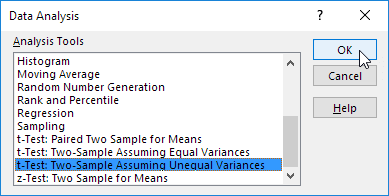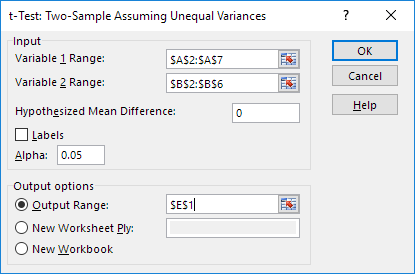# t-Test

This example teaches you how to perform a t-Test in Excel. The t-Test is used to test the null hypothesis that the means of two populations are equal.

Below you can find the study hours of 6 female students and 5 male students.

H0: μ1 – μ2 = 0

H1: μ1 – μ2 ≠ 0To perform a t-Test, execute the following steps.

1. First, perform an F-Test to determine if the variances of the two populations are equal. This is not the case.

2. On the Data tab, in the Analysis group, click Data Analysis.3. Select t-Test: Two-Sample Assuming Unequal Variances and click OK.4. Click in the Variable 1 Range box and select the range A2:A7.

5. Click in the Variable 2 Range box and select the range B2:B6.

6. Click in the Hypothesized Mean Difference box and type 0 (H0: μ1 – μ2 = 0).

7. Click in the Output Range box and select cell E1.8. Click OK.

Result:Conclusion: We do a two-tail test (inequality). lf t Stat < -t Critical two-tail or t Stat > t Critical two-tail, we reject the null hypothesis. This is not the case, -2.365 < 1.473 < 2.365. Therefore, we do not reject the null hypothesis. The observed difference between the sample means (33 – 24.8) is not convincing enough to say that the average number of study hours between female and male students differ significantly.